## SJF Scheduling-

Before you go through this article, make sure that you have gone through the previous article on SJF Scheduling.

In SJF Scheduling,

• Out of all the available processes, CPU is assigned to the process having smallest burst time.
• The main drawback of SJF Scheduling is that it can not be implemented practically.
• This is because burst time of the processes can not be known in advance.

## Techniques to Predict Burst Time-

There are several techniques which try to predict the burst time for the processes so that the algorithm can be implemented.

These techniques are-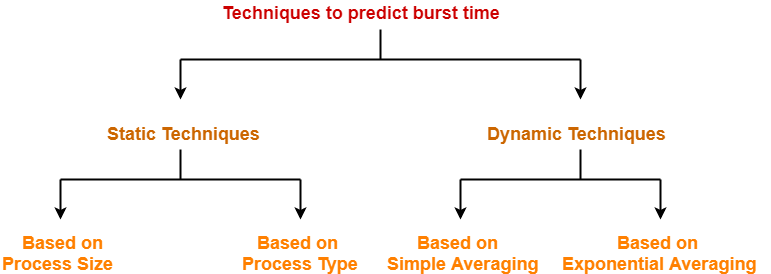## Static Techniques-

There are two static techniques-

1. Based on process size
2. Based on process type

## 1. Based on Process Size-

• This technique predicts the burst time for a process based on its size.
• Burst time of the already executed process of similar size is taken as the burst time for the process to be executed.

## Example-

• Consider a process of size 200 KB took 20 units of time to complete its execution.
• Then, burst time for any future process having size around 200 KB can be taken as 20 units.

### NOTE

• The predicted burst time may not always be right.
• This is because the burst time of a process also depends on what kind of a process it is.

## 2. Based on Process Type-

• This technique predicts the burst time for a process based on its type.
• The following figure shows the burst time assumed for several kinds of processes.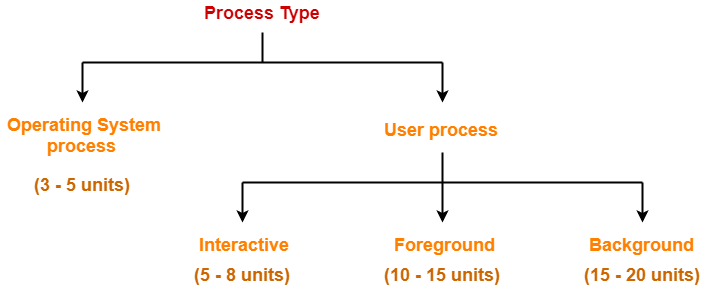## Dynamic Techniques-

There are two dynamic techniques-

1. Based on simple averaging
2. Based on exponential averaging

## 1. Based on Simple Averaging-

• Burst time for the process to be executed is taken as the average of all the processes that are executed till now.
• Given n processes P1, P2, … , Pn and burst time of each process Pi as ti, then predicted burst time for process Pn+1 is given as-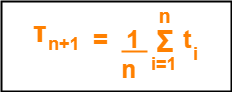## 2. Based on Exponential Averaging-

• Given n processes P1, P2, … , Pn and burst time of each process Pi as ti. Then, predicted burst time for process Pn+1 is given as-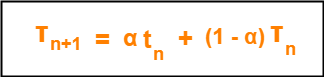where-

• α is called smoothening factor (0<= α <=1)
• tn = actual burst time of process Pn
• Tn = Predicted burst time for process Pn

## Problem-

Calculate the predicted burst time using exponential averaging for the fifth process if the predicted burst time for the first process is 10 units and actual burst time of the first four processes is 4, 8, 6 and 7 units respectively. Given α = 0.5.

## Solution-

Given-

• Predicted burst time for 1st process = 10 units
• Actual burst time of the first four processes = 4, 8, 6, 7
• α = 0.5

### Predicted Burst Time for 2nd Process-

Predicted burst time for 2nd process

= α x Actual burst time of 1st process + (1-α) x Predicted burst time for 1st process

= 0.5 x 4 + 0.5 x 10

= 2 + 5

= 7 units

### Predicted Burst Time for 3rd Process-

Predicted burst time for 3rd process

= α x Actual burst time of 2nd process + (1-α) x Predicted burst time for 2nd process

= 0.5 x 8 + 0.5 x 7

= 4 + 3.5

= 7.5 units

### Predicted Burst Time for 4th Process-

Predicted burst time for 4th process

= α x Actual burst time of 3rd process + (1-α) x Predicted burst time for 3rd process

= 0.5 x 6 + 0.5 x 7.5

= 3 + 3.75

= 6.75 units

### Predicted Burst Time for 5th Process-

Predicted burst time for 5th process

= α x Actual burst time of 4th process + (1-α) x Predicted burst time for 4th process

= 0.5 x 7 + 0.5 x 6.75

= 3.5 + 3.375

= 6.875 units

To gain better understanding about predicting burst time,

Watch this Video Lecture

Next Article-LJF Scheduling | LRTF Scheduling

Get more notes and other study material of Operating System.

Watch video lectures by visiting our YouTube channel LearnVidFun.

## SJF Scheduling-

In SJF Scheduling,

• Out of all the available processes, CPU is assigned to the process having smallest burst time.
• In case of a tie, it is broken by FCFS Scheduling.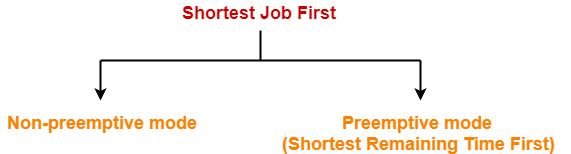• SJF Scheduling can be used in both preemptive and non-preemptive mode.
• Preemptive mode of Shortest Job First is called as Shortest Remaining Time First (SRTF).

• SRTF is optimal and guarantees the minimum average waiting time.
• It provides a standard for other algorithms since no other algorithm performs better than it.

• It can not be implemented practically since burst time of the processes can not be known in advance.
• It leads to starvation for processes with larger burst time.
• Priorities can not be set for the processes.
• Processes with larger burst time have poor response time.

## Problem-01:

Consider the set of 5 processes whose arrival time and burst time are given below-

 Process Id Arrival time Burst time P1 3 1 P2 1 4 P3 4 2 P4 0 6 P5 2 3

If the CPU scheduling policy is SJF non-preemptive, calculate the average waiting time and average turn around time.

## Solution-

### Gantt Chart-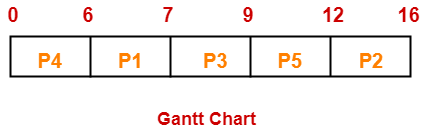Now, we know-

• Turn Around time = Exit time – Arrival time
• Waiting time = Turn Around time – Burst time

 Process Id Exit time Turn Around time Waiting time P1 7 7 – 3 = 4 4 – 1 = 3 P2 16 16 – 1 = 15 15 – 4 = 11 P3 9 9 – 4 = 5 5 – 2 = 3 P4 6 6 – 0 = 6 6 – 6 = 0 P5 12 12 – 2 = 10 10 – 3 = 7

Now,

• Average Turn Around time = (4 + 15 + 5 + 6 + 10) / 5 = 40 / 5 = 8 unit
• Average waiting time = (3 + 11 + 3 + 0 + 7) / 5 = 24 / 5 = 4.8 unit

## Problem-02:

Consider the set of 5 processes whose arrival time and burst time are given below-

 Process Id Arrival time Burst time P1 3 1 P2 1 4 P3 4 2 P4 0 6 P5 2 3

If the CPU scheduling policy is SJF preemptive, calculate the average waiting time and average turn around time.

## Solution-

### Gantt Chart-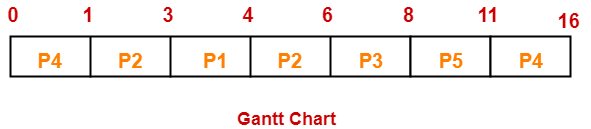Now, we know-

• Turn Around time = Exit time – Arrival time
• Waiting time = Turn Around time – Burst time

 Process Id Exit time Turn Around time Waiting time P1 4 4 – 3 = 1 1 – 1 = 0 P2 6 6 – 1 = 5 5 – 4 = 1 P3 8 8 – 4 = 4 4 – 2 = 2 P4 16 16 – 0 = 16 16 – 6 = 10 P5 11 11 – 2 = 9 9 – 3 = 6

Now,

• Average Turn Around time = (1 + 5 + 4 + 16 + 9) / 5 = 35 / 5 = 7 unit
• Average waiting time = (0 + 1 + 2 + 10 + 6) / 5 = 19 / 5 = 3.8 unit

## Problem-03:

Consider the set of 6 processes whose arrival time and burst time are given below-

 Process Id Arrival time Burst time P1 0 7 P2 1 5 P3 2 3 P4 3 1 P5 4 2 P6 5 1

If the CPU scheduling policy is shortest remaining time first, calculate the average waiting time and average turn around time.

## Solution-

### Gantt Chart-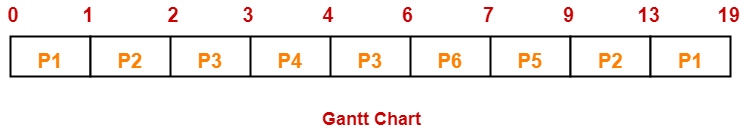Now, we know-

• Turn Around time = Exit time – Arrival time
• Waiting time = Turn Around time – Burst time

 Process Id Exit time Turn Around time Waiting time P1 19 19 – 0 = 19 19 – 7 = 12 P2 13 13 – 1 = 12 12 – 5 = 7 P3 6 6 – 2 = 4 4 – 3 = 1 P4 4 4 – 3 = 1 1 – 1 = 0 P5 9 9 – 4 = 5 5 – 2 = 3 P6 7 7 – 5 = 2 2 – 1 = 1

Now,

• Average Turn Around time = (19 + 12 + 4 + 1 + 5 + 2) / 6 = 43 / 6 = 7.17 unit
• Average waiting time = (12 + 7 + 1 + 0 + 3 + 1) / 6 = 24 / 6 = 4 unit

## Problem-04:

Consider the set of 3 processes whose arrival time and burst time are given below-

 Process Id Arrival time Burst time P1 0 9 P2 1 4 P3 2 9

If the CPU scheduling policy is SRTF, calculate the average waiting time and average turn around time.

## Solution-

### Gantt Chart-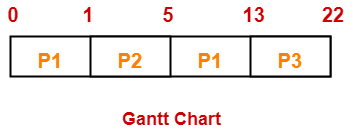Now, we know-

• Turn Around time = Exit time – Arrival time
• Waiting time = Turn Around time – Burst time

 Process Id Exit time Turn Around time Waiting time P1 13 13 – 0 = 13 13 – 9 = 4 P2 5 5 – 1 = 4 4 – 4 = 0 P3 22 22- 2 = 20 20 – 9 = 11

Now,

• Average Turn Around time = (13 + 4 + 20) / 3 = 37 / 3 = 12.33 unit
• Average waiting time = (4 + 0 + 11) / 3 = 15 / 3 = 5 unit

## Problem-05:

Consider the set of 4 processes whose arrival time and burst time are given below-

 Process Id Arrival time Burst time P1 0 20 P2 15 25 P3 30 10 P4 45 15

If the CPU scheduling policy is SRTF, calculate the waiting time of process P2.

## Solution-

### Gantt Chart-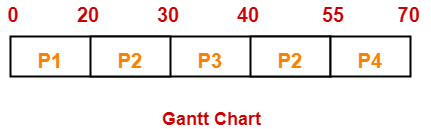Now, we know-

• Turn Around time = Exit time – Arrival time
• Waiting time = Turn Around time – Burst time

Thus,

• Turn Around Time of process P2 = 55 – 15 = 40 unit
• Waiting time of process P2 = 40 – 25 = 15 unit

## Implementation of Algorithm-

• Practically, the algorithm can not be implemented but theoretically it can be implemented.
• Among all the available processes, the process with smallest burst time has to be selected.
• Min heap is a suitable data structure where root element contains the process with least burst time.
• In min heap, each process will be added and deleted exactly once.
• Adding an element takes log(n) time and deleting an element takes log(n) time.
• Thus, for n processes, time complexity = n x 2log(n) = nlog(n)

To gain better understanding about SJF Scheduling,

Watch this Video Lecture

Next Article-Techniques to Predict Burst Time

Get more notes and other study material of Operating System.

Watch video lectures by visiting our YouTube channel LearnVidFun.